# Test Prep Plan - Take a practice test

Take this practice test to check your existing knowledge of the course material. We'll review your answers and create a Test Prep Plan for you based on your results.
How Test Prep Plans work
1
2Based on your results, we'll create a customized Test Prep Plan just for you!
3Study smarter
Study more effectively: skip concepts you already know and focus on what you still need to learn.

# FTCE Middle Grades Math: Circles & Arc of a Circle Chapter Exam

Exam Instructions:

Choose your answers to the questions and click 'Next' to see the next set of questions. You can skip questions if you would like and come back to them later with the yellow "Go To First Skipped Question" button. When you have completed the practice exam, a green submit button will appear. Click it to see your results. Good luck!

### Page 1

#### Question 2 2. In the pictured circle, the measure of angle ACB is 22 degrees and the measure of angle BXD is 120 degrees. What is the measure of angle CAD?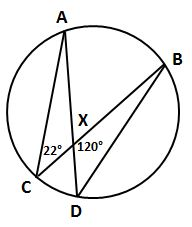#### Question 4 4. If angle ACB is 34 degrees, what is the measure of angle ADB?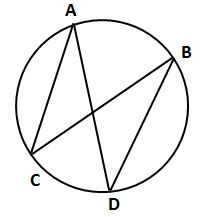#### Question 5 5. If arc BC is 84 degrees, what is the measure of angle ACB?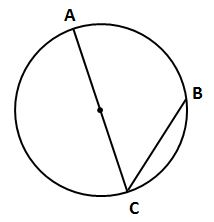### Page 2

#### Question 6 6. In the picture below, if major arc BC is 258 degrees and minor arc BC is 102 degrees, what is the measure of angle BAC?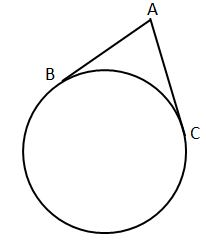#### Question 10 10. In the picture below, if AB is 7, BC is 19 and AD is 8, what is the length of DE?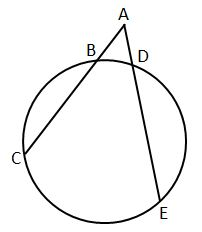### Page 3

#### Question 13 13. If arc AC is 106 degrees and arc BC is 82 degrees, what is the measure of angle ACB?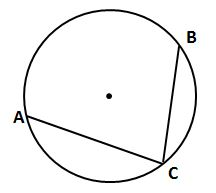### Page 4

#### Question 17 17. In the picture below, if arc CE is 172 degrees and arc BD is 80 degrees, what is the measure of angle CAE?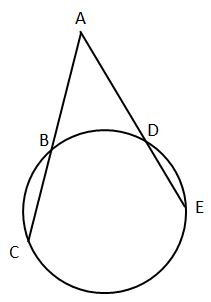#### Question 18 18. In the picture below, if AE is 10, AB is 14 and ED is 16, what is the length of CE?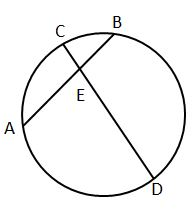#### Question 19 19. In the picture below, if arc AC is 146 degrees and arc BD is 32 degrees, what is the measure of angle AEC?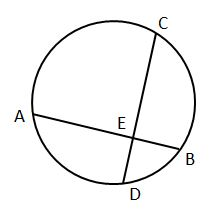### Page 5

#### Question 23 23. In the picture below, if AC is 14 and AB is 10, what is the length of AD?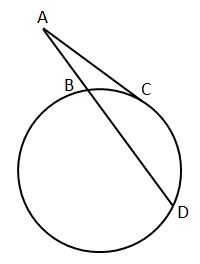### Page 6

#### Question 28 28. If the measure of central angle AOB is 62 degrees, what is the measure of inscribed angle ACB?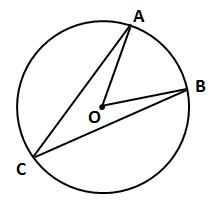#### FTCE Middle Grades Math: Circles & Arc of a Circle Chapter Exam Instructions

Choose your answers to the questions and click 'Next' to see the next set of questions. You can skip questions if you would like and come back to them later with the yellow "Go To First Skipped Question" button. When you have completed the practice exam, a green submit button will appear. Click it to see your results. Good luck!

Support